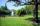# Heron's formula - 8th grade (13y) - math problems

The Heron's formula is used to calculate the contents of a general triangle using the lengths of its sides. Heron's formula states that the area of a triangle whose sides have lengths a, b, and c is:
$S= \sqrt {s(s-a)(s-b)(s-c)}$
, where
$s=\dfrac {a+b+c}{2}$
is the semiperimeter (half perimeter) of the triangle .

#### Number of problems found: 16

• GardensThe area of the square garden is 3/4 of the area of the triangular garden with sides of 80 m, 50 m, 50 m. How many meters of the fence do we need to fence a square garden?
• Sss triangleCalculate the area and heights in the triangle ABC by sides a = 8cm, b = 11cm, c = 12cm
• Is right triangle or notIf right triangle ABC, have sides a=13, b=11.5, c=22.5. Find area.
• Sides ratioCalculate the circumference of a triangle with area 84 cm2 if a:b:c = 10:17:21
• Cardboard boxWe want to make a cardboard box shaped quadrangular prism with rhombic base. Rhombus has a side of 5 cm and 8 cm one diagonal long. The height of the box to be 12 cm. The box will be open at the top. How many square centimeters cardboard we need, if we ca
• ParkIn the newly built park will be permanently placed a rotating sprayer irrigation of lawns. Determine the largest radius of the circle which can irrigate by sprayer P so not to spray park visitors on line AB. Distance AB = 55 m, AP = 36 m and BP = 28 m.
• DiamondRhombus has side 17 cm and and one of diagonal 22 cm long. Calculate its area.
• PrismCalculate the volume of the rhombic prism. Base of prism is rhombus whose one diagonal is 47 cm and the edge of the base is 28 cm. The edge length of the base of the prism and height is 3:5.
• Triangle ABCCalculate the sides of triangle ABC with area 1404 cm2 and if a: b: c = 12:7:18
• DescribedCalculate perimeter of the circle described by a triangle with sides 478, 255, 352.
• Heron backlawCalculate missing side in a triangle with sides 17 and 34 and area 275.
• TriangleCalculate heights of the triangle ABC if sides of the triangle are a=75, b=84 and c=33.
• TrapezoidCalculate area of trapezoid ABCD with sides |AD|= 68 cm, |DC|=35 cm, |CB|=12 cm, |AB|=35 cm..
• TriangleDetermine whether a triangle can be formed with the given side lengths. If so, use Heron's formula to find the area of the triangle. a = 158 b = 185 c = 201
• Annulus from triangleCalculate the content of the area bounded by a circle circumscribed and a circle inscribed by a triangle with sides a = 25mm, b = 29mm, c = 36mm
• One trapeziumOne trapezium has AB=24M, BC=36M, CD=80M, DA=80M long sides. Find the area.

Do you have an interesting mathematical word problem that you can't solve it? Submit a math problem, and we can try to solve it.

We will send a solution to your e-mail address. Solved examples are also published here. Please enter the e-mail correctly and check whether you don't have a full mailbox.

Please do not submit problems from current active competitions such as Mathematical Olympiad, correspondence seminars etc...